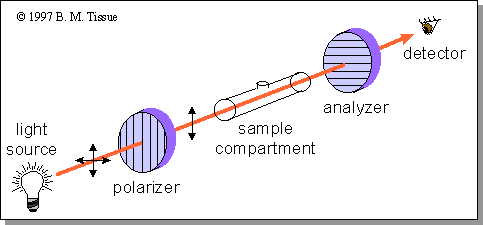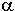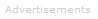# Definition of optical rotation polarimetry

Anisotropic crystalline solids, and samples containing an excess of one enantiomer of a chiral molecule, can rotate the orientation of plane-polarized light. Such substances are said to have optical activity . Measurement of this change in polarization orientation is called polarimetry, and the measuring instrument is called a polarimeter. These measurements are useful for studying the structure of anisotropic materials, and for checking the purity of chiral mixtures. A sample that contains only one enantiomer of a chiral molecule is said to be optically pure. The enantiomer that rotates light to the right, or clockwise when viewing in the direction of light propagation, is called the dextrorotatory (d) or (+) enantiomer, and the enantiomer that rotates light to the left, or counterclockwise, is called the levorotatory (l) or (-) enantiomer.

Optical rotation occurs because optically active samples have different refractive indices for left- and right-circularly polarized light . Another way to make this statement is that left- and right-circularly polarized light travel through an optically active sample at different velocities. This condition occurs because a chiral center has a specific geometric arrangement of four different substituents, each of which has a different electronic polarizability. Light travels through matter by interacting with the electron clouds that are present. Left-circularly polarized light therefore interacts with an anisotropic medium differently than does right-circularly polarized light .

Linearly or plane-polarized light is the superposition of equal intensities of left- and right-circularly polarized light . As plane-polarized light travels through an optically active sample, the left- and right-circularly polarized components travel at different velocities. This difference in velocities creates a phase shift between the two circularly polarized components when they exit the sample. Summing the two components still produces linearly polarized light, but at a different orientation from the light entering the sample. Instrumentation

The simplest polarimeter consists of a monochromatic light source, a polarizer, a sample cell, a second polarizer, which is called the analyzer, and a light detector. The analyzer is oriented 90o to the polarizer so that no light reaches the detector.

Schematic of a polarimeterWhen an optically active substance is present in the beam, it rotates the polarization of the light reaching the analyzer so that there is a component that reaches the detector. The angle that the analyzer must be rotated to return to the minimum detector signal is the optical rotation,.

The amount of optical rotation depends on the number of optically active species through which the light passes, and thus depends on both the sample path length and the analyte concentration. Specific rotation, [], provides a normalize quantity to correct for this dependence, and is defined as:[] = ------ l * d whereis the measured optical rotation in degrees, l is the sample path length in decimeters (dm), and d is the density if the sample is pure liquid, or the concentration if the sample is a solution. In either case, the units of d are g/cm3.

The optical rotatory dispersion (ORD) is the optical rotation as a function of wavelength. It is recorded using a spectropolarimeter, which has a tungsten lamp and a scanning monochromator as the light source. A motorized mount rotates the analyzer to maintain a minimum signal at the detector. Usually a modulation is introduced into the polarization angle of the light beam, so that a DC signal to the analyzer motor then keeps the detector signal centered at the minimum value.Search the Dictionary for More Terms